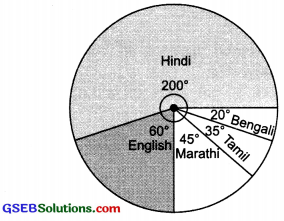# GSEB Solutions Class 8 Maths Chapter 5 Data Handling Ex 5.2

Gujarat Board GSEB Textbook Solutions Class 8 Maths Chapter 5 Data Handling Ex 5.2 Textbook Questions and Answers.

## Gujarat Board Textbook Solutions Class 8 Maths Chapter 5 Data Handling Ex 5.2

Question 1.
A survey was made of find the type of music that a certain group of young people liked in a city. Adjoining pie chart shows the findings of this survey?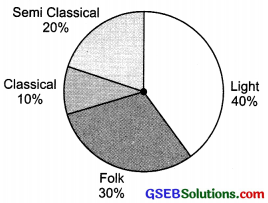From this pie chart answer the following:

1. If 20 people liked classical music, how many young people were surveyed?
2. Which type of music is liked by the maximum number of people?
3. If a cassette company wants to make 1000 CD’s how many of each type would they make?

Solution:
1. Let the required number of young people = x
∴ 10% 0f x = 20
or $$\frac{10}{100}$$ × x = 20 or x = $$\frac{20×100}{10}$$ = 200

2. Maximum number of people like the light music.

3. Total number of CD’s = 1000
∴ Number of CD’s for semi classical
= 20% of 1000
= $$\frac{20}{100}$$ × 1000 = 200
Number of CD’s for classical
= 10% of 1000 = $$\frac{10}{100}$$ × 1000 = 100
Number of CD’s for folks
= $$\frac{30}{100}$$ × 1000 = 300
Number of CD’s for light music
= 40% of 1000 = $$\frac{40}{100}$$ × 1000 = 400Question 2.
A group of 360 people were asked to vote for their favourite season from the three seasons rainy, winter and summer?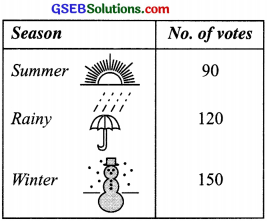1. Which season got the most votes?
2. Find the central angle of each sector?
3. Draw a pie chart to show this information?

Solution:
1. Number of votes are maximum (150) for the winter season.

2. Total votes = 90 + 120 + 150 = 360
∴ Central angle of the sector corresponing to:
Summer season = $$\frac{90}{360}$$ × 360° = 90°
Rainy season = $$\frac{120}{360}$$ × 360° = 120°
Winter season = $$\frac{150}{360}$$ × 360° = 150°

3.Question 3.
Draw a pie chart showing the following information. The table shows the colours preferred by a group of people?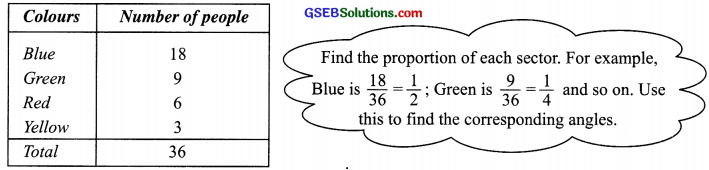Solution:
Central angle of the sector corresponding to:
(a) The blue colour = $$\frac{18}{36}$$ × 360°
= 18 × 10 = 180°

(b) The green colour = $$\frac{9}{36}$$ × 360°
= 90°

(c) The red colour = $$\frac{6}{36}$$ × 360° = 60°

(d) The yellow colour = $$\frac{3}{36}$$ × 360° = 30°
Thus, the required pie chart is given below: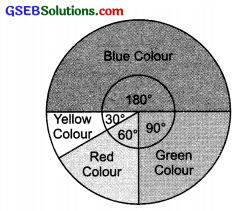Question 4.
The adjoining pie chart gives the marks scored in an examination by a student in Hindi, English, Mathematics, Social Science and Science. If the total marks obtained by the student were 540, answer the following questions?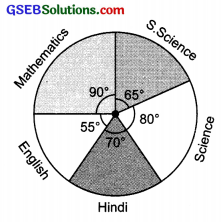1. In which subject did the student score 105 marks?
Hint: For 540 marks, the central angle = 360°. So, for 105 marks, what is the central angle?
2. How many more marks were obtained by the student in Mathematics than in Hindi?
3. Examine whether the sum of the marks obtained in Social Science and Mathematics is more than that in Science and Hindi. Hint: Just study the central angles?

Solution:
1. Total marks = 540
∴ Central angle corresponding to 540 marks = 360°
Central angle corresponding to 105 marks
= $$\frac{360}{540}$$ × 105° = 70°
Since the sector having central angle 70° is corresponding to Hindi.
Thus, the student obtained 105 marks in Hindi.

2. ∵ The central angle corresponding to the sector of Mathematics = 90°
∴ Marks obtained in Mathematics
= $$\frac{90}{360}$$ × 540° = 135
Thus, marks more in Mathematics than in Hindi
= 135 – 105 = 30

3. Since, the sum of the central angles for Social Science and Mathematics
= 65° + 90° = 155°
Also, the sum of the central angles for Science and Hindi
= 80° + 70° = 150°

∵ Marks obtained are proportional to the central angles corresponding to various items and 155° > 150°.
∴ Marks obtained in Science and Mathematics are more than the marks obtained in Science and Hindi.Question 5.
The number of students in a hostel, speaking different languages is given below. Display the data in a pie chart?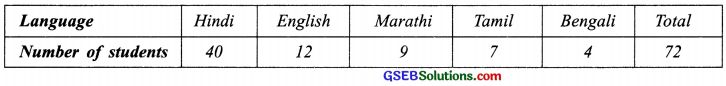Solution:
Central angle of the sector representing:
(a) Hindi language = $$\frac{40}{72}$$ × 360° = 40 × 5° = 200°
(b) English language = $$\frac{12}{72}$$ × 360° = 60°
(c) Marathi language = $$\frac{9}{72}$$ × 360° = 45°
(d) Tamil language = $$\frac{7}{72}$$ × 360° = 35°
(e) Bengali language = $$\frac{4}{72}$$ × 360° = 4 × 5 = 20°
Thus, the required pie chart is given below: